JavaScript实现角距離：中心/重心/点距离/x与y距离/垂线与距离

前言

「角距，即角距离，也称为角分离、视距离、或视分离，在数学(特别是几何学和三角学)和自然科学(包括天文学、地质学等等)，从不同于两个点物体的位置（即第三点）观察这两个物体，由观测者指向这两个物体的直线之间所夹角度的大小。角距离(或分离)与角度本身是同义的，但意义却是对两个天体(对恒星，是当从地球观测)之间线距离的建议(通常是很大或未知的)。」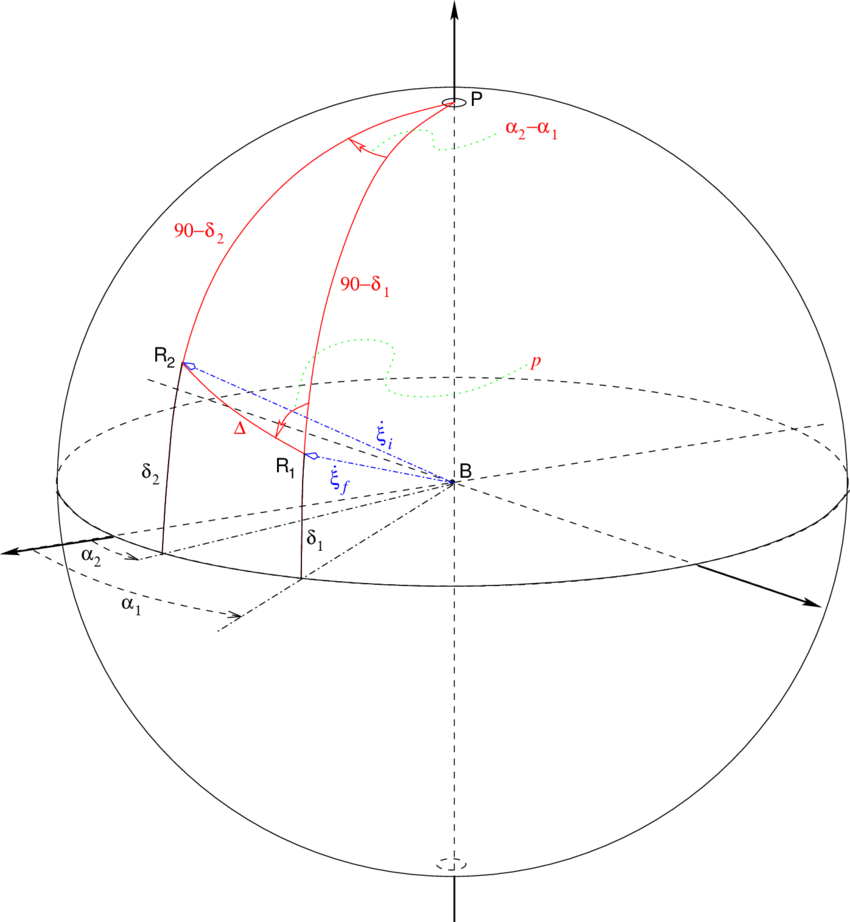ここからやるよ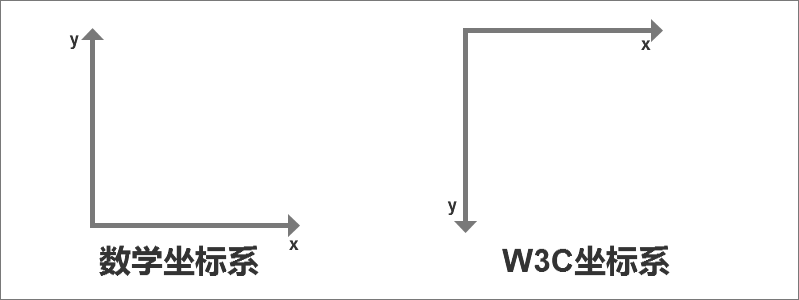canvas的坐标系是左上角（0,0）开始，所以都是正值，负值是看不见的，和中学时候学习的坐标系略有不同。

<!DOCTYPE html>
<html>
<meta charset="utf-8">
<title>Canvas绘制直线</title>
<body>
<canvas id="canvas" width="200" height="150" style="border: 0.0625rem;"></canvas>
</body>
<script>
var canvas = document.getElementById("canvas");
var cxt = canvas.getContext("2d");
//画直线
cxt.moveTo(80,20);
cxt.lineTo(20,60);
cxt.stroke();
}
</script>
</html>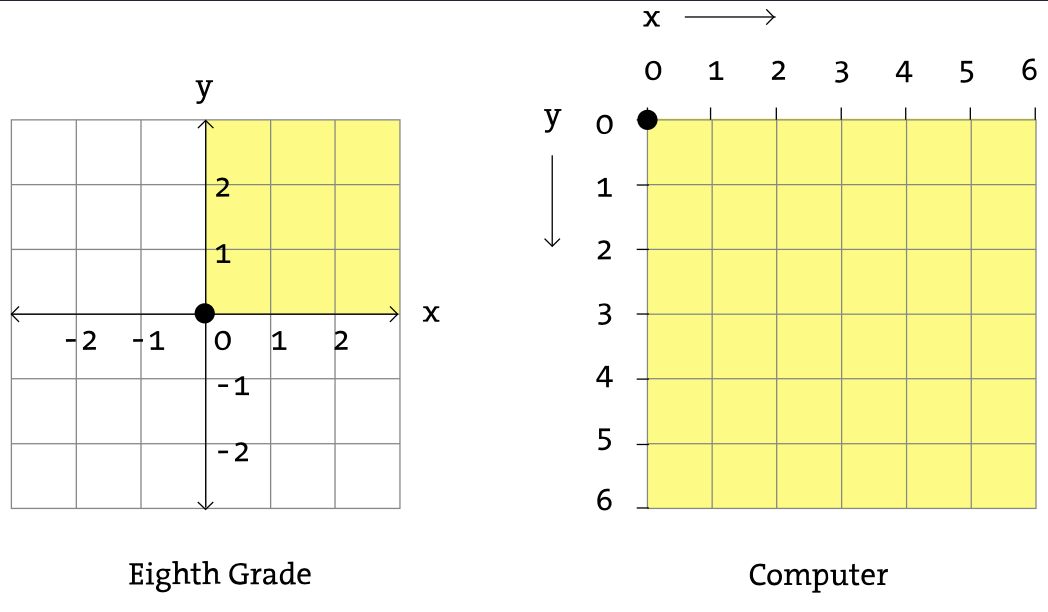1. 中心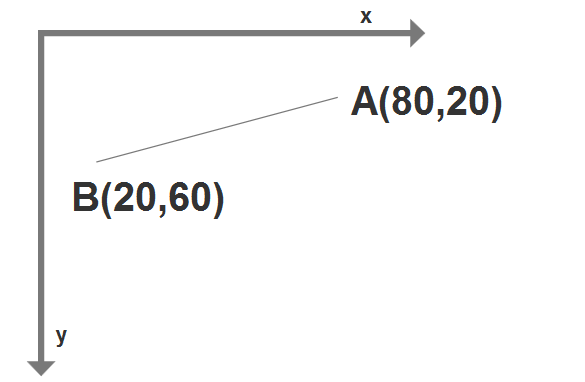//A点
var Ax = 80,
Ay = 20;

//B点
var Bx = 20,
By = 60;

//中心点
var Cx = Ax-Bx,
Cy = Ay-By;

2. 重心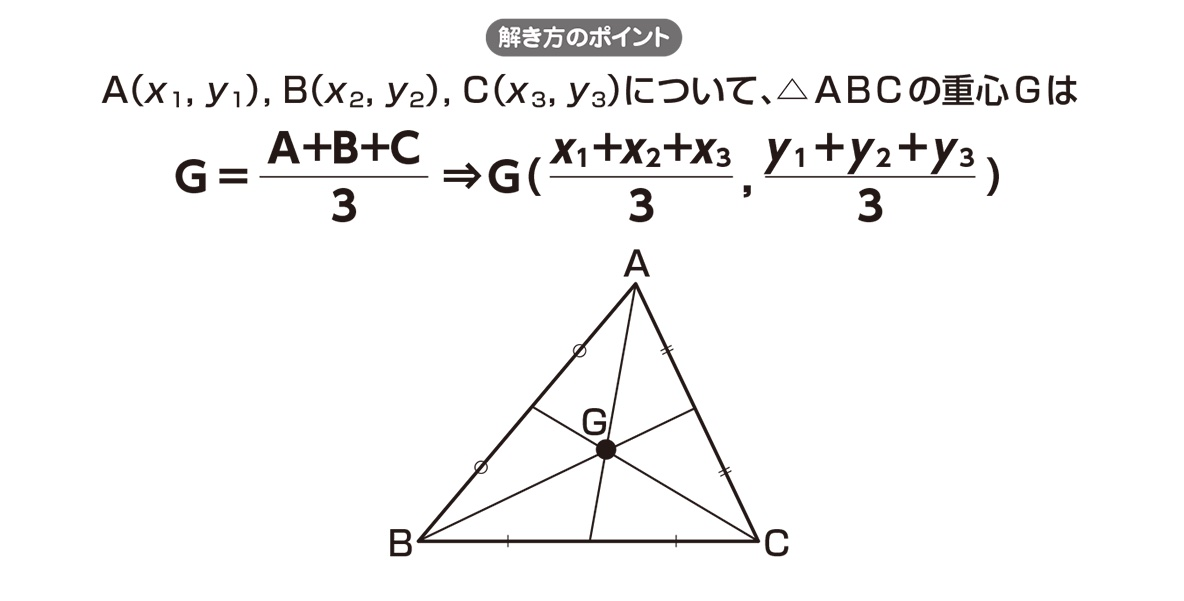var Ax,Ay, //设置A点坐标
Bx,By, //设置B点坐标
Cx,Cy, //设置C点坐标
CGx, //Center of gravity
CGy; //重心
CGx = (Ax + Bx + Cx) / 3;
CGy = (Ay + By + Cy) / 3;

3. 两点距離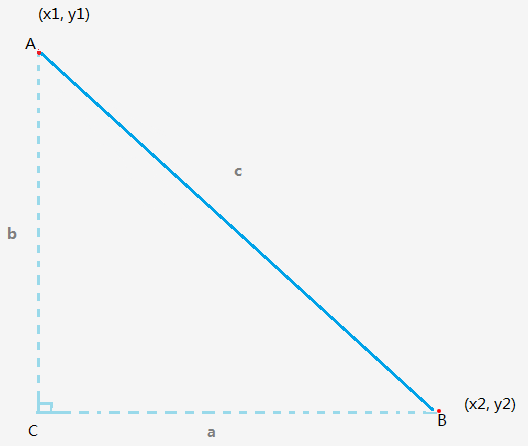function getDistanceBetweenTwoPoints(x1, y1, x2, y2){
var a = x1 - x2;
var b = y1 - y2;

// c^2 = a^2 + b^2
// a^2 = Math.pow(a, 2)
// b^2 = Math.pow(b, 2)
var result = Math.sqrt(Math.pow(a, 2) + Math.pow(b, 2));

return result;
}

Math.sqrt方法是求平方根，Math.pow(n, 2)是求一个数的平方。上面的的方法就是根据两点的坐标求得两点的距离。

4. xy座標距離

① x座標距離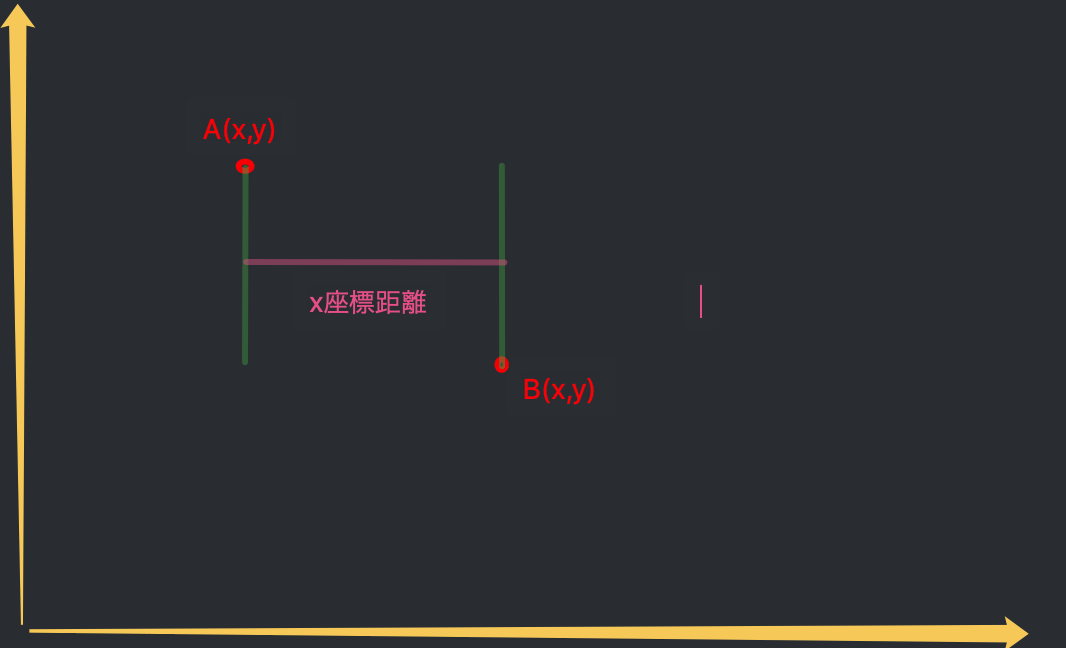var Ax,Ay, //设置A点坐标
Bx,By, //设置B点坐标
Distancex, //距離x
Distancey; //距離y
Distancex = Ax - Bx;
Distancey = Ay - By;

② y座標距離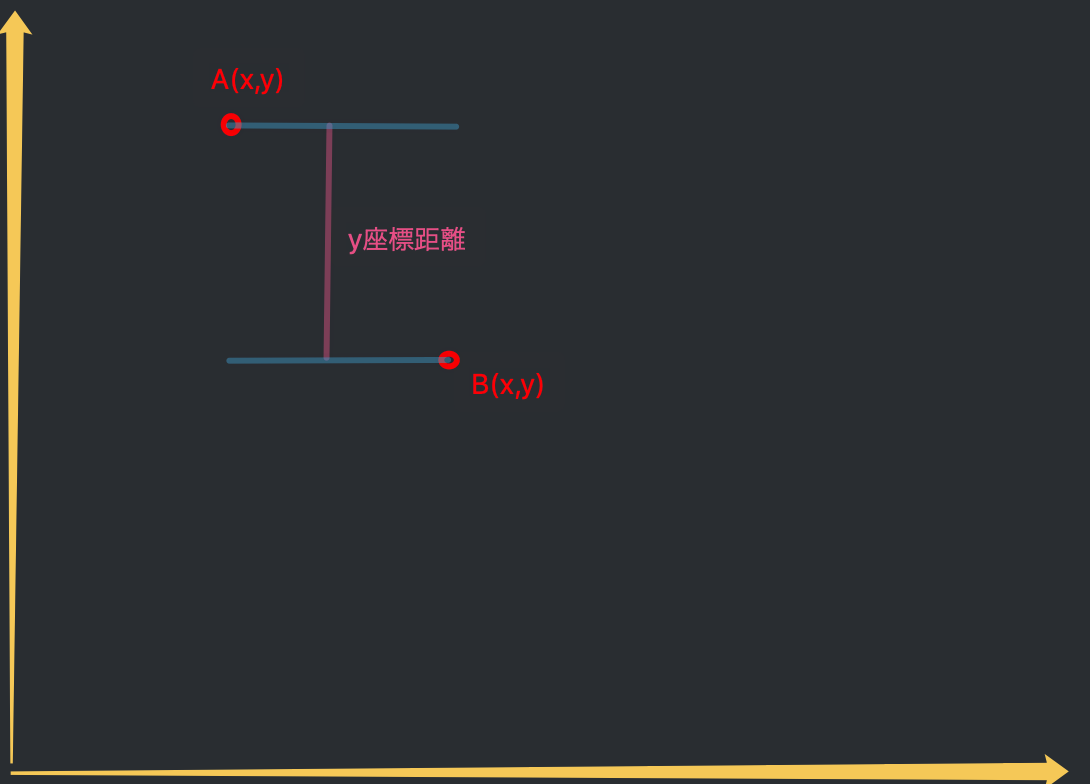var Ax,Ay, //设置A点坐标
Bx,By, //设置B点坐标
Distancex, //距離x
Distancey; //距離y
Distancex = Ax - Bx;
Distancey = Ay - By;

5. 直線の交叉点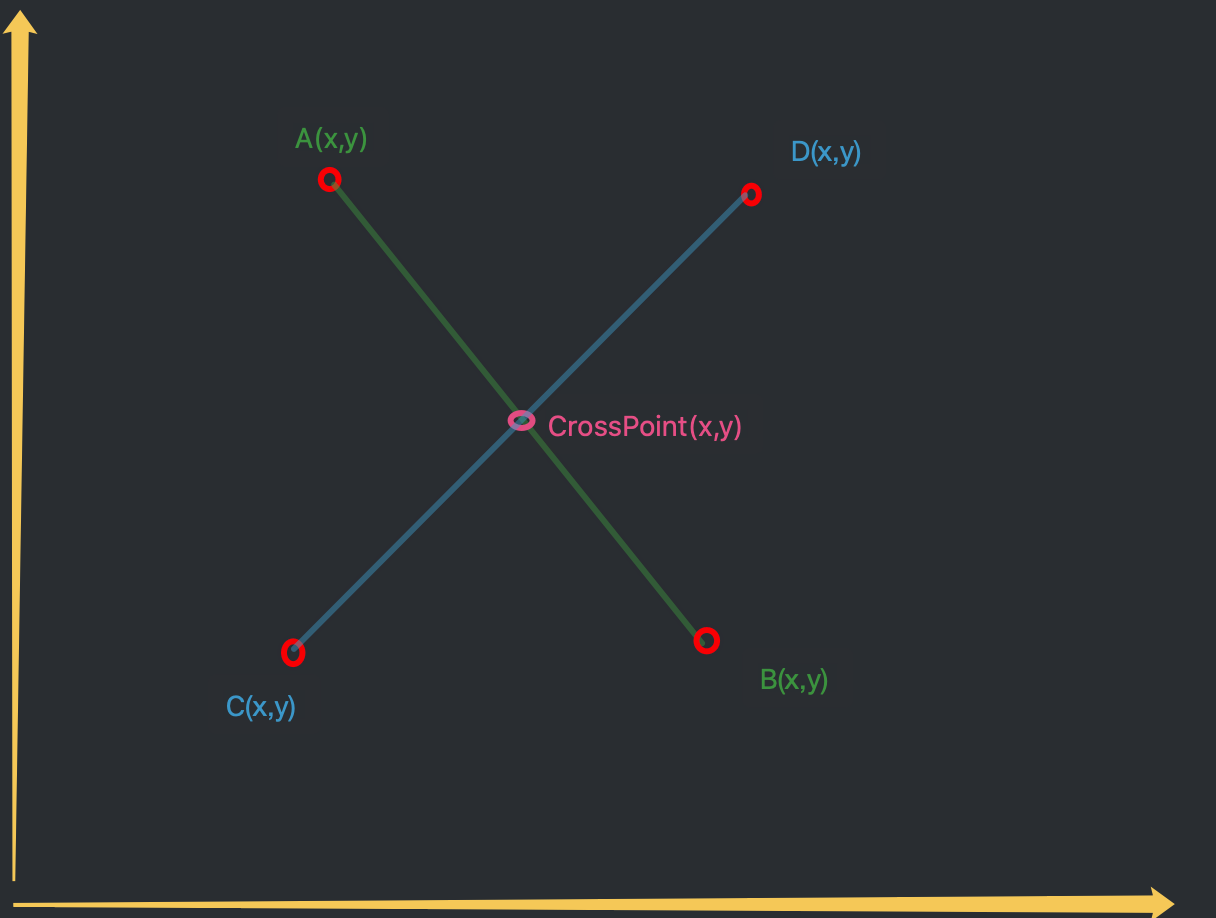$$CrossPointy=\frac{[(Ay-By)\times(Cy-Dy)\times Ax+(Cy-Dy)\times(Bx-Ax)\times Ay + (By-Ay)\times(Cy-Dy)\times Dx+(Dx-Cx)\times(By-Ay)\times Dy]}{[(Bx-Ax)\times(Cy-Dy)+(Ay-By)\times(Cx-Dx)]}$$

$$CrossPointx=Dx+\frac{(Cx-Dx)\times(CrossPointy-Dy)}{(Cy-Dy)}$$

//先求出CrossPointy
CrossPointy = ( (Ay-By)*(Cy-Dy)*Ax + (Cy-Dy)*(Bx-Ax)*Ay + (By-Ay)*(Cy-Dy)*Dx + (Dx-Cx)*(By-Ay)*Dy ) / ( (Bx-Ax)* (Cy-Dy) + (Ay-By)*(Cx-Dx) );
//求CrossPointx
CrossPointx = Dx + (Cx-Dx)*(CrossPointy-Dy) / (Cy-Dy);

6. 点直線間垂線と距離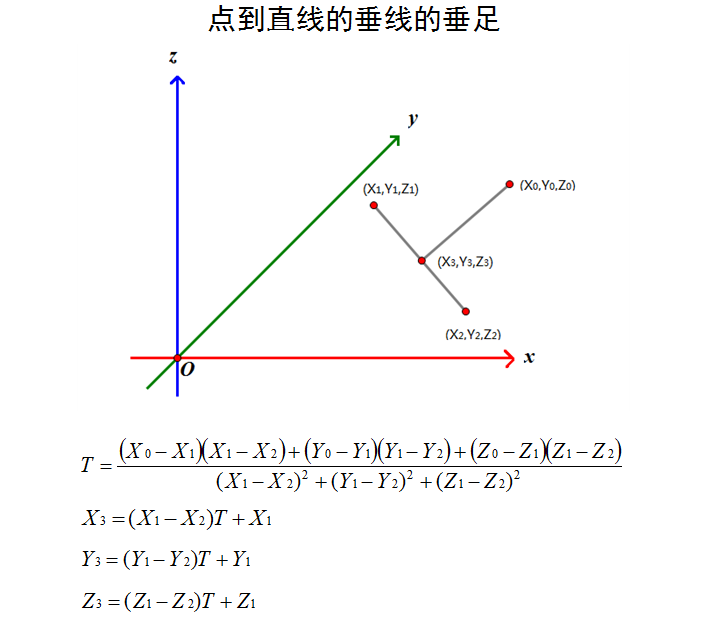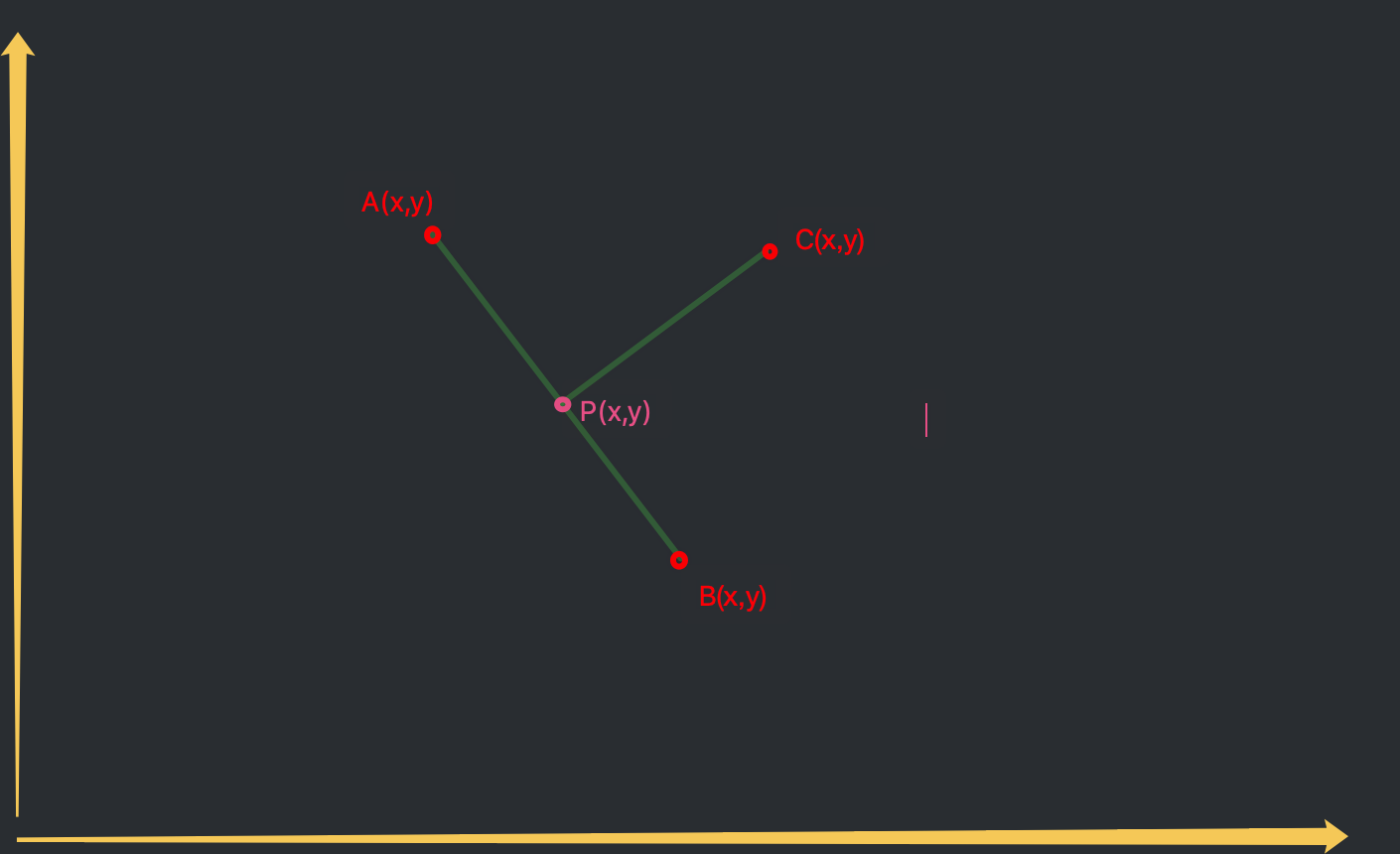$$T=\frac{(C-A)\times(A-B)}{(A-B)^2}$$

$$P=(A-B)\times T+A$$

var Ax,Ay, //设置A点坐标
Bx,By, //设置B点坐标
Cx,Cy, //设置C点坐标
Px,Py,
T;

① 点直線間垂線

//垂线就是 C点 和 P点
T = ( (Cx-Bx)*(Ax-Bx) + (Cy-By)*(Ay-By) ) / ( (Ax-Bx)*(Ax-Bx) + (Ay-By)*(Ay-By) );
Px= (Ax-Bx)*T + Ax;
Py= (Ay-By)*T + Ay;

②点直線間距離

var a = Cx - Px;
var b = Cy - Py;
var Distance = Math.sqrt(Math.pow(a, 2) + Math.pow(b, 2));

Scroll to Top